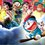# Simply!!

Let $AN$ be the surface of the lake and $O$ be the point of observation such that $OA=h$ meters.

Let $P$ be the position of the cloud and $B$ be its reflection in the lake.

$\text{Then PN = BN}$

$\angle POM = \alpha , \angle BOM = \beta$

$\text{Let } PM = x$

$PN = PM + MN = PM + OA = h + x$

$\text{In}$ $\Delta ABC,$

$\tan\alpha = \dfrac{PQ}{QM} = \dfrac{x}{AN}$

$\implies AN = x.\cot\alpha...............\boxed{1}$

$\text{In}$ $\Delta OMB$

$\tan\beta = \dfrac{DM}{OM} = \dfrac{h+2x}{AN}$

$\implies AN = (x+2h)\cot\beta.............\boxed{2}$

$\text{Equating}$ $\boxed{1}$ $\text{and}$ $\boxed{2}$

$\implies x\cot\alpha = (x+2h)\cot\beta$

$\implies x(\cot\alpha - \cot\beta) = 2h\cot\beta$

$\implies x(\dfrac{1}{\tan\alpha} - \dfrac{1}{\tan\beta}) = \dfrac{2h}{\tan\beta}$

$\implies \dfrac{x(\tan\beta - \tan\alpha)}{\tan\alpha . \tan\beta} = \dfrac{2h}{\tan\beta}$

$\implies x=\dfrac{2h\tan\alpha}{\tan\beta - \tan\alpha}$

$\text{Now height of the cloud is given by PN = x+h}$

$\implies \text{Height of Cloud} = \dfrac{2h\tan\alpha}{\tan\beta - \tan\alpha} + h$

$\implies \text{Height of Cloud} = \dfrac{2h\tan\alpha + h(\tan\beta - \tan\alpha)}{\tan\beta - \tan\alpha}$

$\implies\boxed{\boxed{ \text{Height of Cloud} = \dfrac{h(\tan\alpha + \tan\beta)}{\tan\beta - \tan\alpha}}}$

$\displaystyle \huge{Hence}$ $\huge{Proved}$Note by Sai Ram
4 years, 6 months ago

This discussion board is a place to discuss our Daily Challenges and the math and science related to those challenges. Explanations are more than just a solution — they should explain the steps and thinking strategies that you used to obtain the solution. Comments should further the discussion of math and science.

When posting on Brilliant:

• Use the emojis to react to an explanation, whether you're congratulating a job well done , or just really confused .
• Ask specific questions about the challenge or the steps in somebody's explanation. Well-posed questions can add a lot to the discussion, but posting "I don't understand!" doesn't help anyone.
• Try to contribute something new to the discussion, whether it is an extension, generalization or other idea related to the challenge.
• Stay on topic — we're all here to learn more about math and science, not to hear about your favorite get-rich-quick scheme or current world events.

MarkdownAppears as
*italics* or _italics_ italics
**bold** or __bold__ bold
- bulleted- list
• bulleted
• list
1. numbered2. list
1. numbered
2. list
Note: you must add a full line of space before and after lists for them to show up correctly
paragraph 1paragraph 2

paragraph 1

paragraph 2

[example link](https://brilliant.org)example link
> This is a quote
This is a quote
    # I indented these lines
# 4 spaces, and now they show
# up as a code block.

print "hello world"
# I indented these lines
# 4 spaces, and now they show
# up as a code block.

print "hello world"
MathAppears as
Remember to wrap math in $$ ... $$ or $ ... $ to ensure proper formatting.
2 \times 3 $2 \times 3$
2^{34} $2^{34}$
a_{i-1} $a_{i-1}$
\frac{2}{3} $\frac{2}{3}$
\sqrt{2} $\sqrt{2}$
\sum_{i=1}^3 $\sum_{i=1}^3$
\sin \theta $\sin \theta$
\boxed{123} $\boxed{123}$

## Comments

Sort by:

Top Newest

What is the question that you are answering?

If it's a solution to an existing problem, please post it directly.

Staff - 4 years, 6 months ago

Log in to reply

×

Problem Loading...

Note Loading...

Set Loading...